Next: Sommerfeld Precursor Up: Wave Propagation in Uniform Previous: Wave Propagation in Dispersive

# Wave-Front Propagation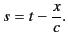(871)

Let us consider the two cases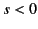and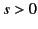separately.

Suppose that. In this case, we distort the path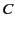, used to evaluate the integral (870), into the pathshown in Figure 8. This is only a sensible thing to do if the real part of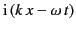is negative at infinity in the upper half-plane. Now, it is clear from the dispersion relation (871) that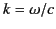in the limit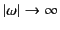. Thus,(872)

It follows thatpossesses a large negative real part along pathprovided that. Thus, Equation (870) yields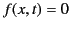(873)

for. In other words, it is impossible for the wave-front to propagate through the dispersive medium with a velocity greater than the velocity of light in a vacuum.

Suppose that. In this case, we distort the pathinto the lower half-plane, because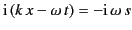has a negative real part at infinity in this region. In doing this, the path becomes stuck not only at the singularity of the denominator at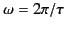, but also at the branch points of the expression for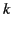. After a little algebra, the dispersion relation (871) yields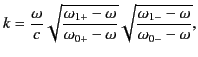(874)

where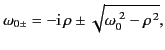(875)

and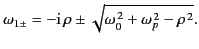(876)

Here,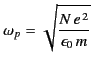(877)

is the plasma frequency, and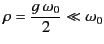(878)

parameterizes the damping. In order to prevent multiple roots of Equation (875), it is necessary to place branch cuts between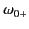and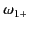, and also between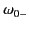and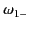. (See Figure 8.)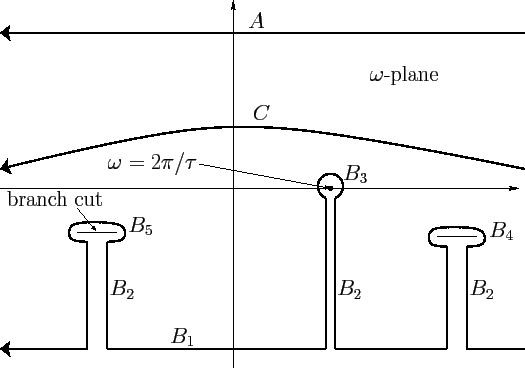The path of integration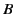is conveniently split into the parts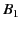through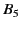. (See Figure 8.) The contribution fromis negligible, because the exponential in Equation (870) is vanishingly small on this part of the integration path. Likewise, the contribution from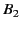is zero, because its two sections always cancel one another. The contribution from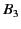follows from the residue theorem: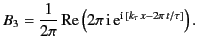(879)

Here,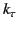denotes the value ofobtained from the dispersion relation (871) in the limit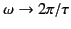. Thus,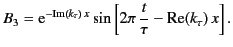(880)

In general, the contributions from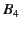andcannot be simplified further. For the moment, we denote them as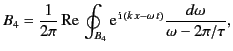(881)

and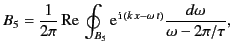(882)

where the paths of integration circle the appropriate branch cuts. Altogether, we have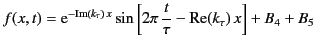(883)

for.

Let us now look at the special case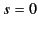. For this value of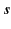, we can change the original path of integration to one at infinity in either the upper or the lower half plane, because the integrand vanishes in each case, through no longer exponentially, but rather as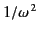. We can see this from Equation (867), which can be written in the form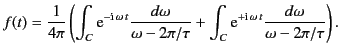(884)

Substitution of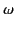for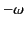in the second integral yields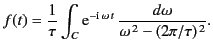(885)

Now, applying dispersion theory, we obtain from the previous equation, just as we obtained Equation (870) from Equation (867),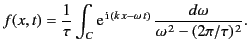(886)

Clearly, the integrand vanishes as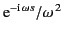in the limit that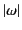becomes very large. Thus, it vanishes asfor. Because we can calculate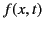using either pathor path, we conclude that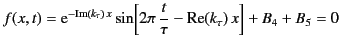(887)

for. Thus, there is continuity in the transition from the regionto the region.

We are now in a position to make some meaningful statements regarding the behavior of the signal at depth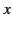within the dispersive medium. Prior to the time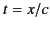, there is no wave motion. In other words, even if the phase velocity is superluminal, no electromagnetic signal can arrive earlier than one propagating at the velocity of light in vacuum,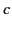. The wave motion for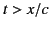is conveniently divided into two parts: free oscillations and forced oscillations. The former are given by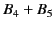, and the latter by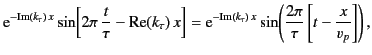(888)

where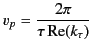(889)

is termed the phase velocity. The forced oscillations have the same sine wave characteristics and oscillation frequency as the incident wave. However, the wave amplitude is diminished by the damping coefficient, although, as we have seen, this is generally a negligible effect unless the frequency of the incident wave closely matches one of the resonant frequencies of the dispersive medium. The phase velocity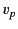determines the velocity at which a point of constant phase (e.g., a peak or trough) of the forced oscillation signal propagates into the medium. However, the phase velocity has no effect on the velocity at which the forced oscillation wave-front propagates into the medium. This latter velocity is equivalent to the velocity of light in vacuum,. The phase velocitycan be either greater or less than, in which case peaks and troughs either catch up with or fall further behind the wave-front. Of course, peaks can never overtake the wave-front.

It is clear from Equations (876), (877), (882), and (883) that the free oscillations oscillate with real frequencies that lie somewhere between the resonant frequency,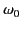, and the plasma frequency,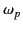. Furthermore, the free oscillations are damped in time like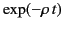. The free oscillations, like the forced oscillations, begin at time. At, the free and forced oscillations exactly cancel one another [see Equation (888)]. As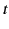increases, both the free and forced oscillations set in, but the former rapidly damp away, leaving only the forced oscillations. Thus, the free oscillations can be regarded as some sort of transient response of the medium to the incident wave, whereas the forced oscillations determine the time asymptotic response. The real frequency of the forced oscillations is that imposed externally by the incident wave, whereas the real frequency of the free oscillations is determined by the nature of the dispersive medium, quite independently of the frequency of the incident wave.

One slightly surprising result of the previous analysis is the prediction that the signal wave-front propagates into the dispersive medium at the velocity of light in vacuum, irrespective of the dispersive properties of the medium. Actually, this is a fairly obvious result. As is well described by Feynman in his famous Lectures on Physics, when an electromagnetic wave propagates through a dispersive medium, the electrons and ions that make up that medium oscillate in sympathy with the incident wave, and, in doing so, emit radiation. The radiation from the electrons and ions, as well as the incident radiation, travels at the velocity. However, when these two radiation signals are superposed, the net effect is as if the incident signal propagates through the dispersive medium at a phase velocity that is different from. Consider the wave-front of the incident signal, which clearly propagates into the medium with the velocity. Prior to the arrival of this wave-front, the electrons and ions are at rest, because no information regarding the arrival of the incident wave at the surface of the medium can propagate faster than. After the arrival of the wave-front, the electrons and ions are set into motion, and emit radiation which affects the apparent phase velocity of radiation that arrives somewhat later. But this radiation certainly cannot affect the propagation velocity of the wave-front itself, which has already passed by the time the electrons and ions are set into motion (because of their finite inertia).Next: Sommerfeld Precursor Up: Wave Propagation in Uniform Previous: Wave Propagation in Dispersive
Richard Fitzpatrick 2014-06-27